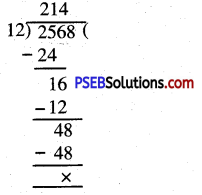# PSEB 5th Class Maths Solutions Chapter 2 Fundamental Operations on Numbers Intext Questions

Punjab State Board PSEB 5th Class Maths Book Solutions Chapter 2 Fundamental Operations on Numbers InText Questions and Answers.

## PSEB 5th Class Maths Solutions Chapter 2 Fundamental Operations on Numbers InText Questions

Try These : (Textbook Page No.27)

Question 1.
Solve.

(a)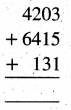Solution: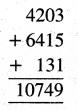(b)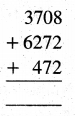Solution: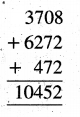(c)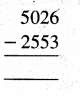Solution:(d)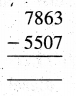Solution: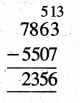Question 2.
Fill in the blanks :
(a) 115 + 327 = 327 + ____
(b) 321 + 0 = ___
(c) 139 × 1 = ___
(d) 625 × 0 = ___
(e) 339 – 0 = ___
(f) 119 ÷ 119 = ___
(g) 128 ÷ 16 = ___
(h) 720 + 500 = ___
(i) 10000 ÷ 10 = ___
(j) 152 ÷ 19 = ___
Solution:
(a) 115 + 327 = 327 + 115
(b) 321 + 0 = 321
(c) 139 × 1 = 139
(d) 625 × 0 = 0
(e) 339 – 0 = 339
(f) 119 ÷ 119 = 1
(g) 128 ÷ 16 = 8
(h) 720 + 500 = 1220
(i) 10000 ÷ 10 = 100
(j) 152 ÷ 19 = 8

Question 3.
Let’s Do : 19 = 1

(a) In a school, there are 342 boys and 369 girls. How many total number of students are there in the school ?
Solution: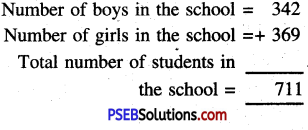(b) In a godown, there are 459 bags of wheat and 813 bags of rice. How many bags are there in total ?
Solution: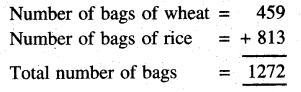(c) In a year, Harmanpreet Kaur scored 1790 runs and Mitali Raj scored 1299 runs. How many more runs were scored by Harmanpreet Kaur than Mitali Raj ?
Solution:
Harmanpreet Kaur scored runs = 1790
Mitali Raj scored runs = 1299
Number of more runs’ scored by Harmanpreet Kaur than Mitali Raj = 1790 – 1299 = 491.

(d) Harpreet took f 10000 from his father and bought a bicycle for ₹ 3540. How much amount is left with him ?
Solution: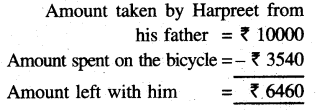(e) A shopkeeper has 625 packets of toffees. In each packet, there are 100 toffees. How many toffees in total the shopkeeper has ?
Solution:
Number of packets = 625
Number of toffees in each packet =100
Total number of toffees shopkeeper has = 625 × 100 = 62500.

(f) There is 250 litre diesel, in the diesel tank of a truck. It covers 9 km distance with one litre of diesel. How much distance can be covered with the diesel ?
Solution:
Quantity of diesel in the tank = 250 litres
Distance covered with one litre of diesel = 9 kmTotal distance covered with the diesel = 9 × 250 km = 2250 km.(g) In a school, there are 648 students. 18 students can sit in a school van to go for a picnic. How many vans are required to take all the students to picnic ?
Solution:
Total number of students in the school = 648
Number of students that can sit in a van = 18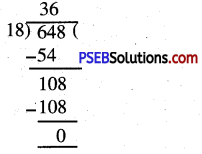Number of vans required = 648 ÷ 18
= 36.

(h) In a garden, there are 2568 guava trees. If there are 12 trees in a row then how many rows are there for 2568 guava trees ?
Solution:
Number of guava trees in the garden = 2568
Number of guava trees in a row = 12
Total number of TOWS = 2568 ÷ 12
= 214